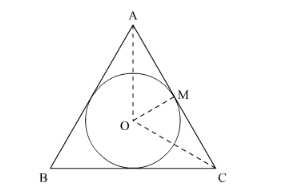# The area of incircle of an equilateral triangle is 154 cm2

Question:

The area of incircle of an equilateral triangle is $154 \mathrm{~cm}^{2}$. The perimeter of the triangle is

(a) 71.5 cm

(b) 71.7 cm

(c) 72.3 cm

(d) 72.7 cm

Solution:

Area of incircle of equilateral triangle is $154 \mathrm{~cm}^{2}$We have to find the perimeter of the triangle. So we will use area to get,

Area of incircle $=154$

$\pi r^{2}=154$

$r=\sqrt{\frac{154}{\pi}} \mathrm{cm}$

As triangle is equilateral so,

$\angle \mathrm{OCM}=30^{\circ}$

So,

$\tan 30^{\circ}=\frac{r}{\mathrm{MC}}$

$\mathrm{MC}=\sqrt{\frac{154(3)}{\pi}} \mathrm{cm}$

So,

$\mathrm{AC}=2(\mathrm{MC})$

$=2\left(\sqrt{\frac{154(3)}{\pi}}\right) \mathrm{cm}$

Therefore perimeter of the triangle is,

$=3(\mathrm{AC})$

$=6\left(\sqrt{\frac{462}{3.14}}\right)$

$=72.7 \mathrm{~cm}$